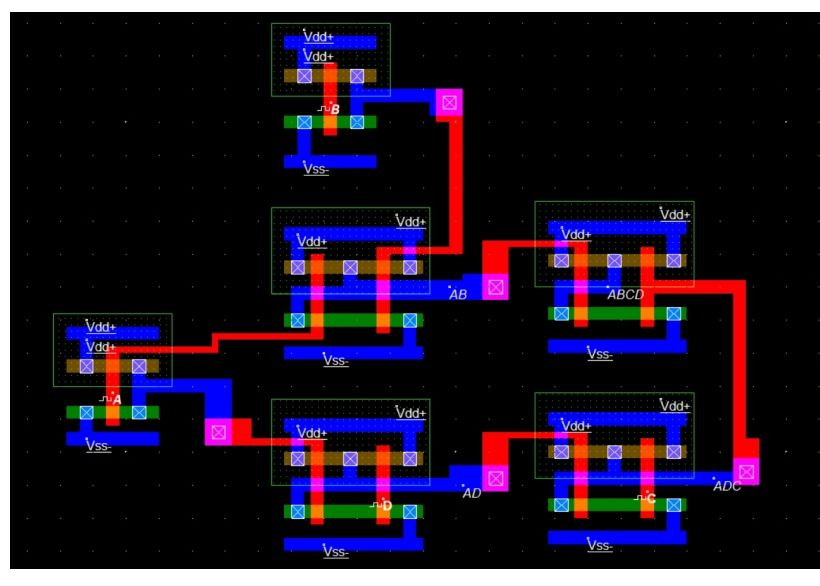No result found.

# Designing Cmos circuit from Boolean expressions (Microwind)Akshay Mishra29 May, 2020View Full Project arrow_forward

Outline

• Designing Cmos circuit from Boolean expressions.

Introduction

A Boolean expression is a logical statement that is either TRUE and FALSE. The logic symbols “0” and “1” being used to represent a digital input or output, we can also use them as constants for a permanently “Open” or “Closed” circuit or contact respectively. A set of rules or laws of Boolean Algebra expressions have been invented to help reduce the number of logic gates needed to perform a particular logic operation resulting in a list of functions or theorems known commonly as the laws of Boolean Algebra. Boolean Algebra is the mathematics we use to analyse digital gates and circuits. we can use these “Laws of Boolean” to both reduce and specify a complex Boolean expression in an attempt to reduce the number of logics based on logic that has its own set of rules or laws which are used to define and reduce Boolean expression. The variables used in Boolean Algebra only have one of two possible values, a logic “0”and a logic “1” but an expression can have an infinite number of variables all labelled individually to represent inputs to the expression. Most of Boolean expression use AND, OR and NOT operator. A Boolean expression can consist of Boolean data, such as the following:  Boolean values  Boolean variables of formulas  Functions that yield Boolean results  Boolean values calculated by comparison operators

Aim

To design and fabricate Cmos circuits in microwind.

Team
• Akshay Mishra

Microwind

cmosHello users, I am the cofounder of thesocialcomment. If you are reading this, you must be curious about things and we respect that.

Post has no comments. Be the first to comment
View Full Project arrow_forward

Outline

• Designing Cmos circuit from Boolean expressions.